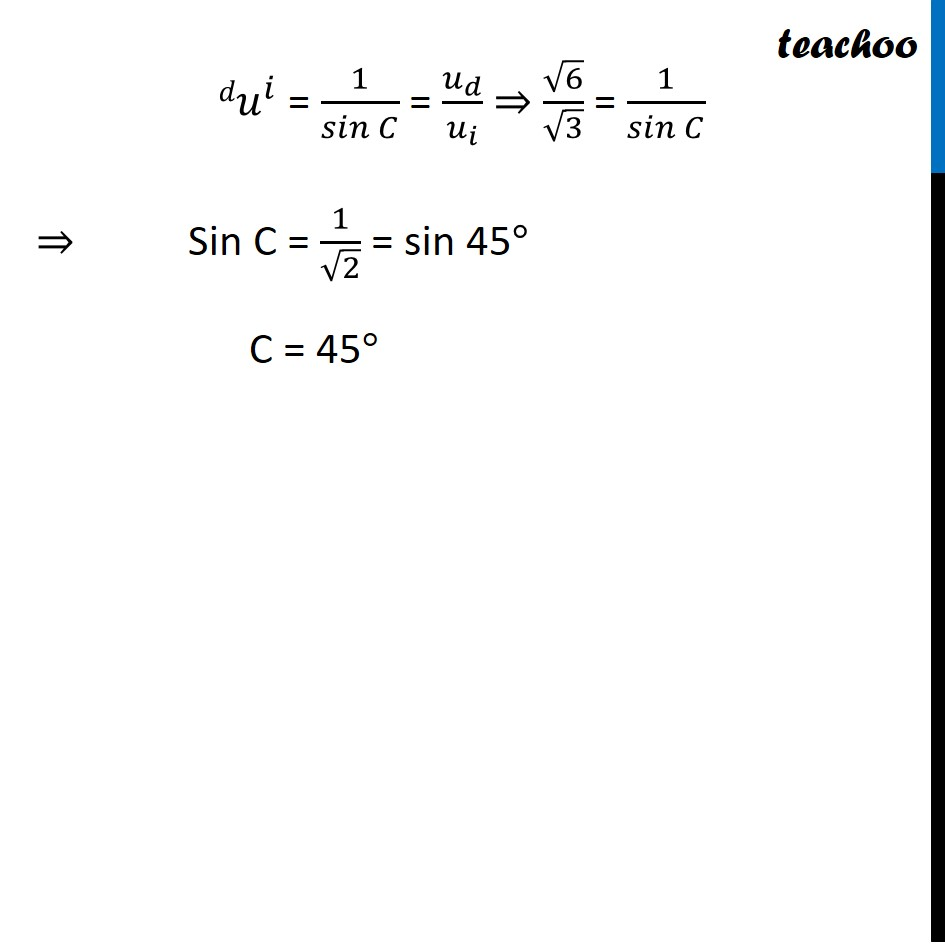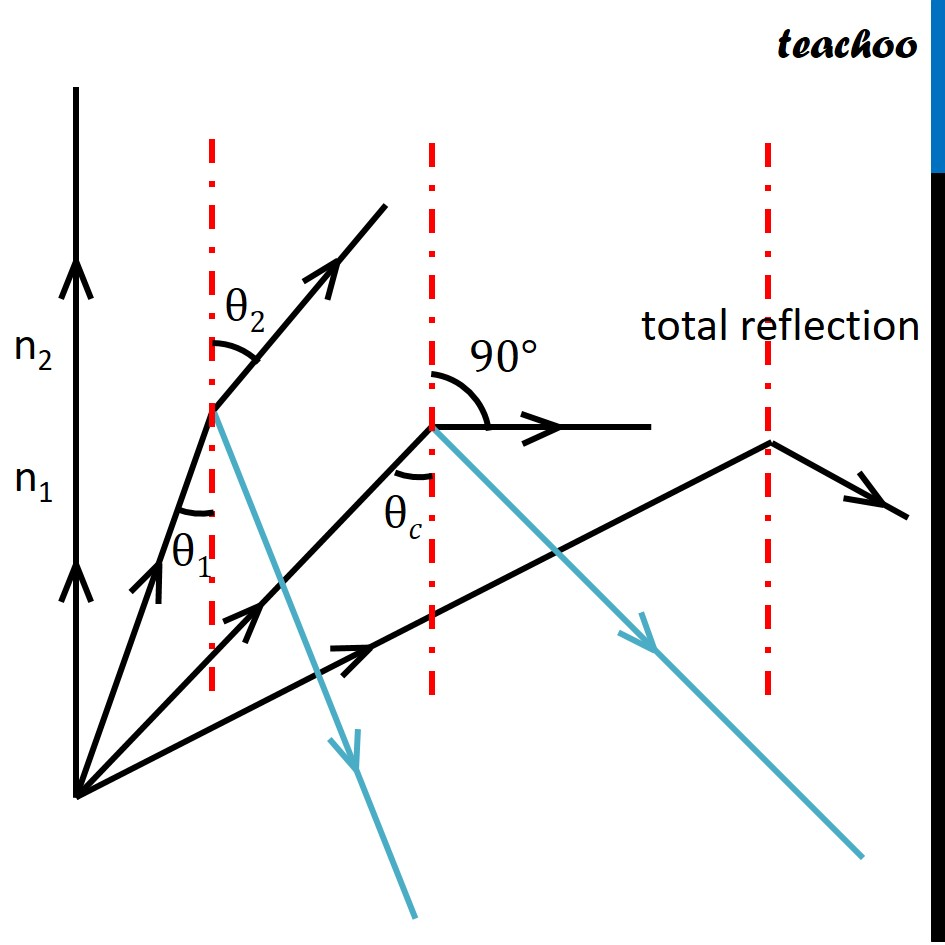Assertion Reasoning Questions (MCQ)

Class 10
Chapter 10 Class 10 - Light - Reflection and Refraction

## Reason (R): μ =  1 / sinC, where μ is the refractive  index of diamond with respect to liquid.

Explanation: when light travels from denser medium to lesser denser medium then total internal reflection occurs.

sin C = 1 / n

• C is the critical angle
• n is the refractive index of denser medium (here, diamond).

Therefore, the Refractive index of diamond w.r.t.  liquidThus, the critical angle is 45 degrees. Therefore, TIR will not take place at 30 °.Thus,

• Assertion is false
• Reason is true

So, the correct answer is (d)

Correct option: (d)

Learn in your speed, with individual attention - Teachoo Maths 1-on-1 Class